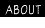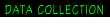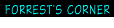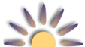[ TERC VHS-1 Sun Photometer ] [ Manual TOC ]

You will use the data from your Langley test to find what's called the extraterrestrial constant, the signal the TERC VHS-1 would give at the top of the atmosphere (4). In other words, if you took the TERC VHS-1 along on a trip into Earth orbit in the Space Shuttle, the signal you would measure using the TERC VHS-1 during a space walk should be very close to what you measure on the ground using the Langley method.

There are several ways to analyze your data to find the extraterrestrial or ET constant, all of which should yield the same result. After you find your instrument's ET constant, you will insert it in a formula that will give you a standardized measurement of haze known as aerosol optical thickness (AOT).

When you made your Langley measurements for a range of Sun angles, each time the angle changed the thickness of the atmosphere (the air mass) between you and the Sun changed (see Fig. 6.). When the Sun is straight overhead, its light passes through one thickness of atmosphere or one air mass. The air mass (m) increases as the Sun moves closer to the horizon. The m for each of your measurements is 1 divided by the sine of the Sun's angle (<) above the horizon or m = 1/sun<.

If you make a graph of the natural logarithm (ln) of the signal you measured versus m when you made the measurement, the data points should fall along a straight line out to an m of 10 or so, assuming the sky conditions were stable during your measurement period. If you extend the straight line, the ET constant will be where the line crosses the air mass axis at m = 0. When m = 0, there is no air mass, which means this is the signal the TERC VHS-1 will give if you could take it to the top of the atmosphere.

Several methods are available for extracting the ET constant from your Langley test data. They should all give similar or nearly identical results. All three methods perform what is called a linear regression on your data. Briefly, a linear regression fits the equation for a straight line, in this case, ln of signal = a + (b x air mass), to your data. You then use this equation to find the signal for m = 0.

The first two methods require that you determine the air mass for each measurement. The TERC VHS-1 spreadsheet does this automatically, and can be downloaded from: ftp://ftp.concord.org/pub/haze/vhs1-download/ The methods given in the Appendix require that you use a calculator to convert the Sun angles to the respective air masses. The air mass is the reciprocal of the Sun's angle above the horizon, so enter the angle and press the SIN key. Then press the 1/x key to get the air mass.

### A. Graph Paper Method

1. Make a table of the TERC VHS-1 measurements for a range of air masses up to about 10. The first column should be the air mass (m) for each measurement and the second column the Sun signal - the dark signal.
2. Add a third column to your table for the natural logarithm (ln) of the signal. (Use a scientific calculator with a ln key.)
3. Print this title on the abscissa (x or horizontal axis) of the graph: Air mass (m).
4. Print this title on the ordinate (y or vertical axis) of the graph: ln of signal.
5. Label the major divisions of the abscissa 0 to 10, as shown in Fig. 7.
6. Plot the pairs of data in the first and third columns on your table on the graph.
7. Use a straight edge to draw a line through the center of the points. Extend the line to the ordinate as shown in Fig. 7.
8. The ET constant is where the line crosses the ordinate. Find the value at this point and write it on your graph as you will need it for the haze formula. The ET value derived from the hand drawn graph in Fig. 7 is based on the first Langley test made with a prototype TERC VHS-1. The ET constant is 1.292, which is very close to the values given by the calculator and a computer program.

### B. Scientific Calculator Method

1. Make a table of the TERC VHS-1 measurements for a range of air masses up to about 10. The first column should be the air mass (m) for each measurement and the second column the Sun signal - the dark signal.
2. Add a third column to your table for the natural logarithm (ln) of the signal. (Use a scientific calculator with a ln key.)
3. Enter the data pairs into a scientific calculator capable of performing a linear regression.
4. Insert the coefficients given by the calculator to calculate the ln of the signal when m = 0. This number is the ET constant, so write it on your table. The ET constant for the prototype TERC VHS-1 is 1.2946.

 [ TERC VHS-1 Sun Photometer ] [ Manual TOC ]Haze-SPAN, A project of the Concord Consortium Copyright © 1997. All rights reserved. Last updated: 21-Apr 1997: http://www.concord.org/haze/analyze.html Questions about Haze-SPAN: carolyn@concord.org | about this site: webmaster@concord.org